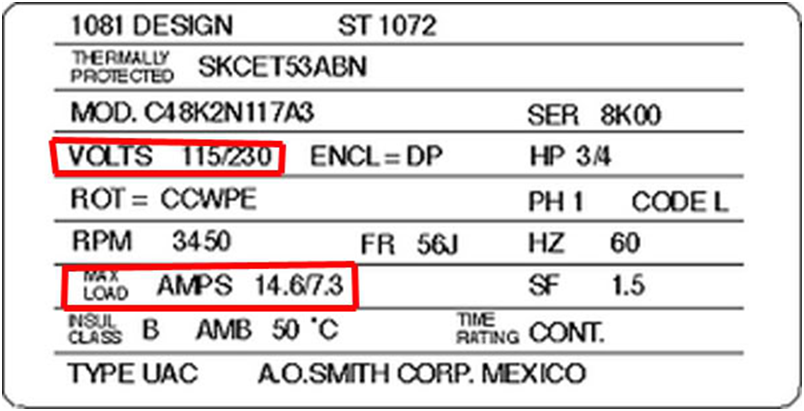# FPU Blog

You can see a one minute video discussing the cost of pool pump operation from Florida Public Utilities Facebook page at the end of this article. This article will give you the tools to figure out the cost of your pool pumps operation. Many pools in Florida have two pumps, one to filter and one to power the vacuum. So, both pumps must be calculated to get your monthly cost.

On your pump motor will be a name plate that looks similar to what is in the picture. The only twopieces of information you need from the name plate is the volts and the amps. You will notice next to volts 115/230 and next to the word AMPS 14.6/7.3. Many pumps can be wired either 115 or 230. As you would think the AMPS listed on the left of the diagonal are for 115 VOLTS and the number on the right is for 230 VOLTS.

VOLTS X AMPS = Watts. 115 VOLTS X 14.6 AMPS = 1679 Watts. There are 1,000 kilowatts in one watt, so we want to divide 1679 Watts by 1,000 = 1.679 Kilowatts. Electricity is billed by kilowatt hours or KWH. Now all you need to know is how many hours your pump runs and what you pay per KWH. Let’s use 6 hours per day for a 30 day billing cycle which equals 180 hours. 180 hours times 1.679 kilowatts = 302.22 KWH. Assume your electric utility charges \$0.14 per KWH. You simply multiply your total kilowatt hours 302.22 X the rate of \$0.14 to discover you will pay \$42.31 for the month to run your pump motor.

Here is the formula with no explanation. VOLTS X AMPS = Watts divided by 1,000 = KW X Hours of operation for the month = KWH X charge per KWH = Cost per month.

For all your energy related questions, contact your energy experts at Florida Public Utilities www.fpuc.com/energyexpert

The Energy Minute

The Cost of Pool Pumps

Posted by Florida Public Utilities on Monday, February 8, 2016

### Comment on This Post

Submit your comment – plus, see what other people are saying.